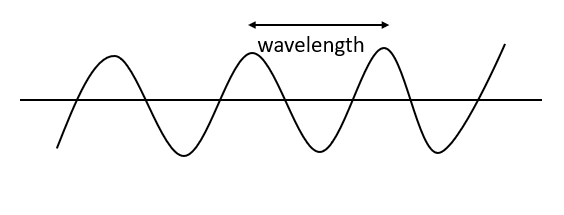Courses
Courses for Kids
Free study material
Offline Centres
MoreLast updated date: 08th Dec 2023
Total views: 281.7k
Views today: 5.81k

# Define wavelength of a wave.Verified
281.7k+ views
Hint: The length between two continuous compressions or two continuous rarefactions is called the wavelength. Wavelength is the length from one trough to another or from one crest to another of a wave, an electromagnetic wave, a sound wave, or any other wave. The crest is the largest point of the wave, whereas the trough is the smallest. Since wavelength is length, it is estimated in lengths such as meters, centimeters, millimeters, nanometres, etc. The wave's Wavelength is the ratio of the speed of the wave to the frequency.

Complete step-by-step solution:
Wavelength can be described as the length between two consecutive crests or troughs of a wave. It is estimated in the wave direction. The wavelength is generally represented by $\lambda$. Its SI unit is meter.Wavelength and frequency are inversely correlated. As the wavelength of a wave increments, its frequency lowers. The equation that relates the two is:
$\lambda = \dfrac{c}{\nu}$
Where, c is the speed of light.
$\nu$ is the frequency.
A wave comprises alternation crests and troughs. The wavelength is described as the distance between two same consecutive points on the waveform. The amplitude is the elevation of the wave. A wave with a tiny wavelength has a large frequency because more waves move a given point in a specific amount of time. A wave with a larger wavelength has a lower frequency.
A wave cycle contains one complete wave—starting at the zero points, going up to a wave crest, moving back down to a wave trough, and returning to the zero points again. The wavelength of a wave is the length between any two identical points on nearby waves. It is simplest to imagine the wavelength of a wave as the length from one wave crest to the succeeding.
The wavelength of light changes with colors, i.e., it is distinctive for every color. Red color has the greatest wavelength while violet has the shortest. The wavelength of UV radiation is lower than violet light. Similarly, the wavelength of infrared radiation is higher than the wavelength of red light.

Note: The repetitive motion characterizes waves. Imagine a toy ship traveling the waves in a pool. As the water wave crosses under the boat, it goes up and down constantly and frequently. While the wave moves horizontally, the boat only goes vertically up and down. Wavelength is inversely proportional to frequency. This means the longer the wavelength, the lower the frequency. In a likewise manner, the shorter the wavelength, the higher the frequency will be.